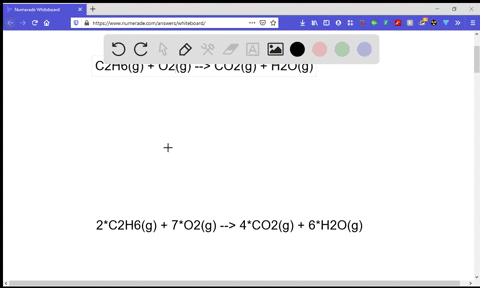Enroll in one of our FREE online STEM summer camps. Space is limited so join now!View Summer Courses### Find $\Delta S_{\mathrm{rxn}}^{\circ}$ for the co…

04:47Ohio State University

Need more help? Fill out this quick form to get professional live tutoring.

Get live tutoring
Problem 34

For each reaction, predict the sign and find the value of $\Delta S_{\mathrm{rxn}}^{\circ}$
(a) $3 \mathrm{NO}_{2}(g)+\mathrm{H}_{2} \mathrm{O}(l) \longrightarrow 2 \mathrm{HNO}_{3}(l)+\mathrm{NO}(g)$
(b) $\mathrm{N}_{2}(g)+3 \mathrm{F}_{2}(g) \longrightarrow 2 \mathrm{NF}_{3}(g)$
(c) $\mathrm{C}_{6} \mathrm{H}_{12} \mathrm{O}_{6}(s)+6 \mathrm{O}_{2}(g) \longrightarrow 6 \mathrm{CO}_{2}(g)+6 \mathrm{H}_{2} \mathrm{O}(g)$

Check back soon!

## Discussion

You must be signed in to discuss.